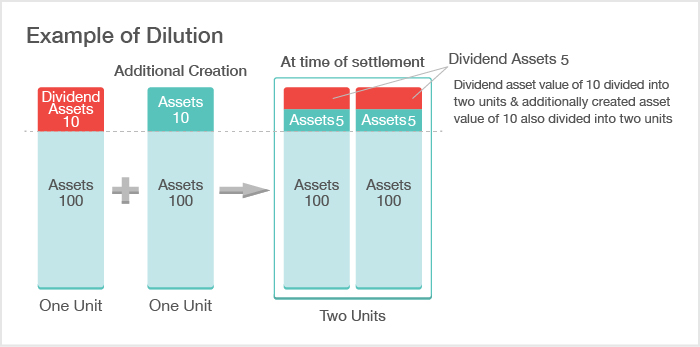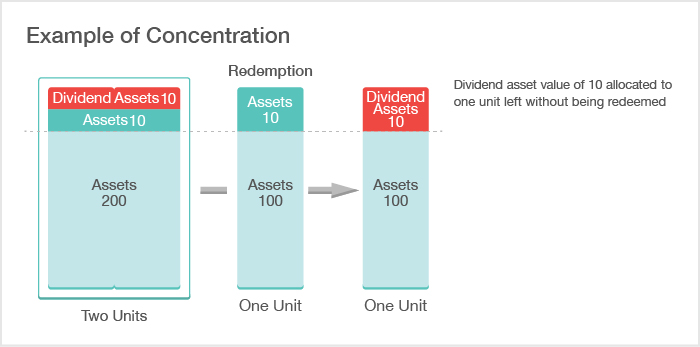• Font Size
• S
• M
• L

# Risks

Investors should be aware of the following risks when investing in ETFs.

## Price Movement Risk

### Movement of Stock Price Index, etc.

There is a possibility the price of the stock price index, etc. may drop due to a variety of economic factors. Due to this, it is possible that an ETF's price will drop and dividends will be reduced. (However, risk is diversified when compared to individual stock investment.)

### Currency Fluctuations

ETFs traded at TSE are all JPY-denominated. When investing in foreign currency-denominated assets, investors need to consider not only factors giving rise to changes in prices, including stock indices, but also currency fluctuations of the relevant currencies and JPY.
For information on foreign exchange risks or currency hedge, please see the webpage indicated below.

## Disparity Risks between Stock Price Indices, etc., Base Value, and Market Price

ETFs are managed by asset management companies so that price movements of underlying stock indices, etc. coincide with those of base values, which indicate the net asset value per unit of ETFs. However, the price movements of stock indices, etc. do not always coincide with those of base values due to such factors as ex-dividend, dividend dilution/concentration, and costs related to the underlying securities, which are described below.

In addition, ETF trading takes into consideration the levels of both stock indices, etc. and base values, etc. Market price movements are ruled by the forces of supply and demand. As such, base values, etc. may not coincide with the price movements of the market. When investing in ETFs, please pay adequate attention to such risks.

For data on disparity, please see the Stock Price Index, Base Value, and Market Price.

### Disparity due to Ex-dividend

Many Japanese companies whose stocks are constituents of stock indices such as TOPIX close their books in March. Record date for rights to the stock dividend falls on around the settlement period. After the record date for rights has been declared, the stock price falls by the amount of the expected dividend. As a result, the price of the relevant stock index also falls.

As for ETFs tracking such stock indices, although the valuation of stocks falls when stocks held are given ex-dividend status, net asset value per unit of ETFs will not fall, because the amount of the expected dividend can be posted as accrued dividend, and the drop in price by the amount of valuation will be compensated. Thus, disparity will temporarily occur between the stock price index and the prices of the ETFs.
Receivable dividends for ETFs will be distributed at the time of settlement. As such, the disparity will be dissolved. (However, in cases of dividend dilution or concentration, which is described later, the disparity may not be dissolved.)
Disparity between ex-dividend date of stocks and that of ETFs will cause the disparity in prices of the relevant stock index and ETF.

For ETFs that invest in stock index futures, including leveraged and inversed types, prices of stock index futures are formed, etc. by deducting the receivable in advance, and there is no ex-dividend on an ex-dividend date like that for stock indices. As such, on the ex-dividend date, the prices of ETFs will be different from those of the stock index that is being tracked.

### Disparity due to Dividend Dilution/Concentration

Concerning ETFs whose dividends are paid at the time of settlement, dividend payout assets will be equally divided and distributed according to the number of outstanding ETFs at the time of settlement. Creation/redemption is conducted for ETFs daily, and the number of ETF issued changes.
Since dividend payout assets accumulated by the time of settlement are distributed according to the number of outstanding ETFs at the time of settlement, due to creation/redemption immediately before the time of settlement, the yield becomes lower (dilution of dividend) or higher (concentration of dividend), which will lead to disparity between stock index and ETF prices.
Dividend dilution turns into a factor of ETF prices exceeding the relevant stock index, while dividend concentration turns into a factor of lower ETF prices than the relevant stock index.

#### Dividend Dilution

Let's assume that a dividend assets value of 10 was accumulated with ETF assets per unit of 100 and net asset value per unit of 110. The dividend amount per unit will be 10 at the time of settlement and dividend. However, if one unit had been additionally created before the settlement, the unit would be created at the price of 110 with net asset value of 220 (assets value of 210 and dividend assets value of 10). (The dividend assets value remains at 10 in the entire fund.)
In this case, as the divided assets value of 10 is divided into two units at the time of settlement and dividend, the dividend amount per unit will be 5. This is called dividend dilution.

The received dividend amount will be 5 instead of 10, which might have seemed to be a loss. However, a net asset value of 210 will be left in the ETF with net assets of 105 per unit. The portion of 5, which will not be distributed as a dividend, will be reserved in the ETF. Thus, it can be said that it reach the break-even point (*).

* However, for investors who conducted additional creation, they pay a net asset value of 110 to obtain one unit of the ETF, receive a dividend amount of 5, and have one unit of the ETF with a net asset value of 105. As the principal will be substantially reimbursed, such investors can see the dividend of 5 as a profit. However, it should be noted that the profit will be subject to taxation.#### Dividend Concentration

Let's assume that a dividend assets value of 10 was built up to an ETF asset value of 210 per two units and net asset value per unit of 110. When one unit is redeemed at a price of 110, an assets value of 110 is paid. Then, the net asset value of the ETF will be 110 (assets value of 100 and dividend assets value of 10). Since the dividend assets value remains at 10, which is the dividend amount per unit at the time of settlement and dividend, the dividend amount per unit at the time of settlement and dividend will be 10. This is called dividend concentration.## Liquidity Risks

When the volume of trading and orders of ETFs are small, trading prices may not reflect actual market conditions of stock indices, etc.

## Credit Risk

Some ETFs invest in linked-bonds and OTC derivatives. Such ETFs also involve credit risk. For details, please refer to the following pages:

## Other Risks

There may be difficulties in tracking the stock price index, etc., when the market experiences sudden shifts. An ETF may be delisted if it falls under the delisting criteria specified by TSE. In cases where the management company deems continuation of the trust difficult, the trust may be concluded.